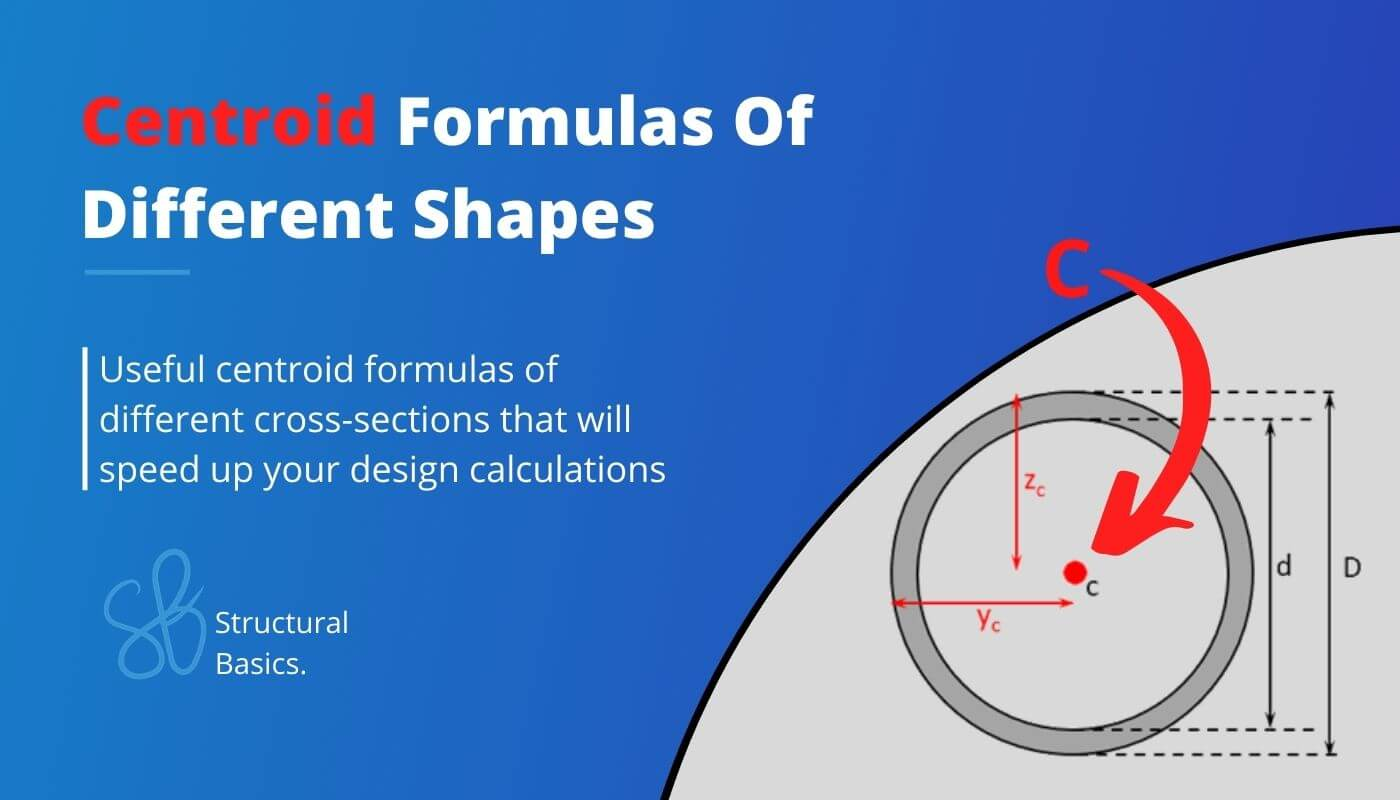# 9 Centroid Formulas Of Different ShapesAre you an engineer, student, or just someone who wants to speed up the calculation of centroid of cross-sections? 🙋‍♂️🙋‍♂️

While it’s very important to know how to calculate the centroid of different shapes, it’s much quicker to use formulas. 🚀🚀

In this article, we’ll show, the most important centroid formulas of cross-sections used in structural engineering.

Alright, let’s get started. 🔥🔥

## Centroid of Rectangular Section

This is probably the most used cross-section in structural engineering.🔥🔥

### Rectangle

#### Vertical Distance

$z_{c} = \frac{h}{2}$

#### Horizontal Distance

$y_{c} = \frac{w}{2}$

## Centroid of Symmetrical I Section

This is the most used cross-section in steel design.🔥🔥

### I or H Profile

#### Vertical Distance

$z_{c} = \frac{h}{2}$

#### Horizontal Distance

$y_{c} = \frac{w_f}{2}$

## Centroid of Unsymmetrical I Section

### Unsymmetrical I or H Profile

#### Vertical Distance

$$z_c = \frac{A_1 \cdot z_1 + A_2 \cdot z_2 + A_3 \cdot z_3}{A_1 + A_2 + A_3}$$

#### Horizontal Distance

$y_{c} = \frac{w_f}{2}$

With,
$A_i$ = Area of the different parts (i = 1, 2, 3)
$c_i$ = Distance from the top edge to the centroids of the different parts (1, 2, 3)

## Centroid of Circular Section

### Circle

#### Vertical Distance

$$z_c = \frac{D}{2}$$

#### Horizontal Distance

$y_{c} = \frac{D}{2}$

## Centroid of Hollow Circular Section

### Hollow Circle

#### Vertical Distance

$$z_c = \frac{D}{2}$$

#### Horizontal Distance

$y_{c} = \frac{D}{2}$

## Centroid of Unsymmetrical Triangle

### Unsymmetrical Triangle

#### Vertical Coordinate zc

$$z_c = \frac{z_1 + z_2 + z_3}{3}$$

#### Horizontal Coordinate yc

$y_{c} = \frac{y_1 + y_2 + y_3}{3}$

## Centroid of Symmetrical Triangle

### Triangle

#### Vertical Distance

$$z_c = \frac{h}{3}$$

#### Horizontal Distance

$y_{c} = \frac{w}{2}$

## Centroid of T Section

### T Profile

#### Vertical Distance

$$z_c = \frac{A_1 \cdot z_1 + A_2 \cdot z_2}{A_1 + A_2}$$

#### Horizontal Distance

$y_{c} = \frac{w_{f.t}}{2}$

With,
$A_i$ = Area of the different parts (i = 1, 2)
$c_i$ = Distance from the top edge to the centroids of the different parts (1, 2)

## Centroid of U Section

### U Profile

#### Vertical Distance

$$z_c = \frac{A_1 \cdot z_{c.1} + A_2 \cdot z_{c.2} + A_3 \cdot z_{c.3}}{A_1 + A_2 + A_3}$$

#### Horizontal Distance

$$y_c = \frac{A_1 \cdot y_{c.1} + A_2 \cdot y_{c.2} + A_3 \cdot y_{c.3}}{A_1 + A_2 + A_3}$$

## Conclusion

Calculating the centroid of a cross-section is usually the first step in calculating the moment of inertia or section modulus. Check out those detailed guides. ⬇️⬇️

In case you already know how to calculate moment of inertia and section modulus, here are formula articles to speed up your design calculations.

I hope that this post helps you speed up your engineering calculations.

In case you still have questions.

Let us know in the comments below. ✍️✍️

## Centroid Formulas FAQ

What are centroid formulas?

Centroid formulas are equations that help you find the coordinates of the centroid of a shape. In other words, the distance from the top, bottom, left and right edges to the centre of mass of a cross-section is calculated.

What do you need to use centroid formulas?

To use a centroid formula, you need to know the dimensions of the cross-section of the structural element. Then you can insert those values into the formula to find the centroid.

What is a centroid?

In short: It’s the center of mass or the geometric center of a 2D shape/cross-section.